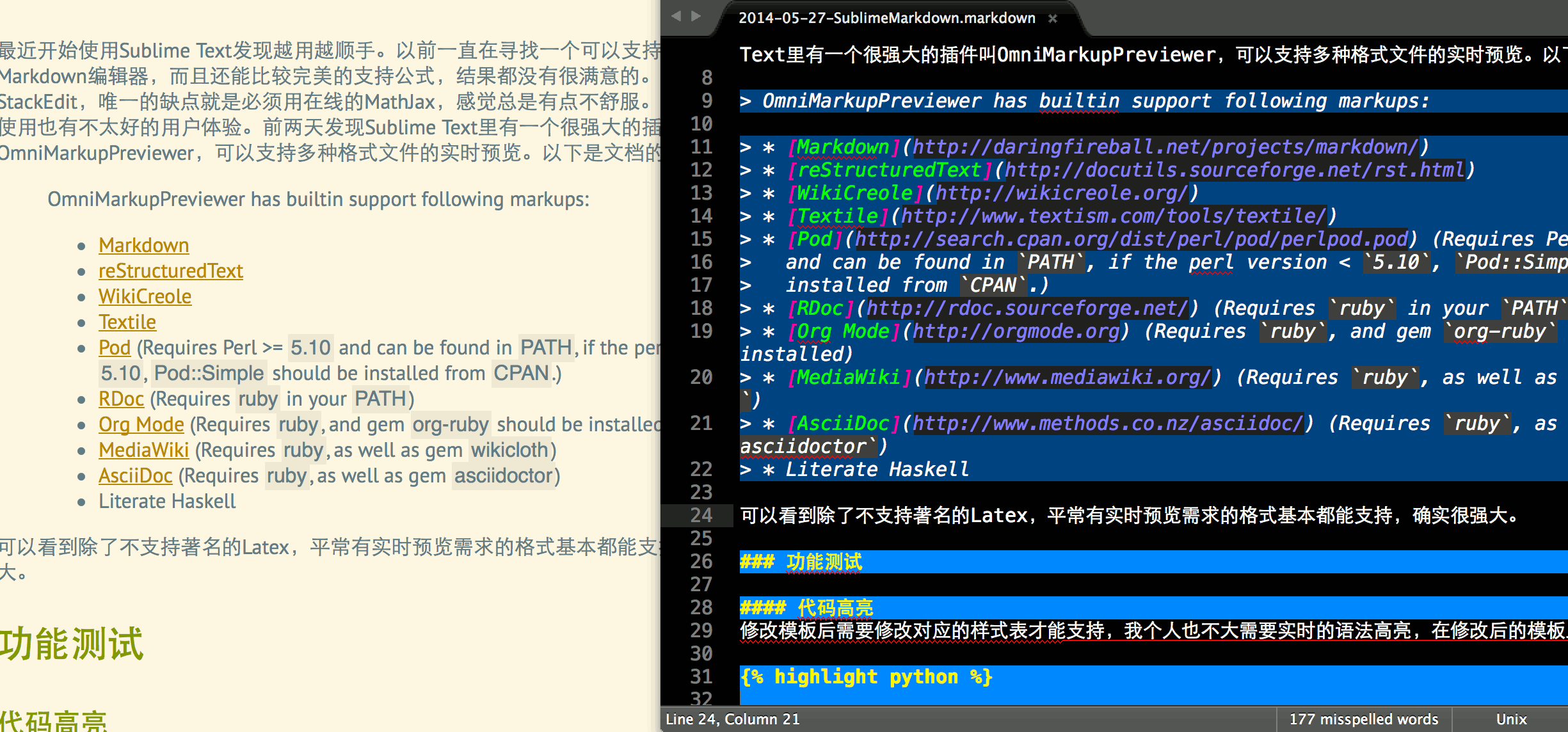# Sublime Text 可视化编辑Markdown最近开始使用Sublime Text发现越用越顺手。以前一直在寻找一个可以支持离线实时预览的Markdown编辑器，而且还能比较完美的支持公式，结果都没有很满意的。后来就开始使用StackEdit，唯一的缺点就是必须用在线的MathJax，感觉总是有点不舒服。当然在浏览器里使用也有不太好的用户体验。前两天发现Sublime Text里有一个很强大的插件叫OmniMarkupPreviewer，可以支持多种格式文件的实时预览。以下是文档的摘要：

OmniMarkupPreviewer has builtin support following markups:

• Markdown
• reStructuredText
• WikiCreole
• Textile
• Pod (Requires Perl >= 5.10 and can be found in PATH, if the perl version < 5.10, Pod::Simple should be installed from CPAN.)
• RDoc (Requires ruby in your PATH)
• Org Mode (Requires ruby, and gem org-ruby should be installed)
• MediaWiki (Requires ruby, as well as gem wikicloth)
• AsciiDoc (Requires ruby, as well as gem asciidoctor)

### 功能测试

#### 实时公式预览

$\begin{array}{l} f(x+2h)-f(x-2h)-2\left(f(x+h)+f(x-h)\right)\\ =4 h \frac{d}{d x} f{\left (x \right )} + \frac{16h^{3}}{6} \frac{d^{3}}{d x^{3}} f{\left (x \right )}-2\left(2 h \frac{d}{d x} f{\left (x \right )} + \frac{2h^{3}}{6} \frac{d^{3}}{d x^{3}} f{\left (x \right )}\right) +\mathcal{O}\left(h^{5}\right)\\ = 2h^3 \frac{d^{3}}{d x^{3}} f{\left (x \right )} +\mathcal{O}\left(h^{5}\right) \end{array}$ $\therefore \frac{d^{3}}{d x^{3}} f{\left (x \right )} =\frac{f(x+2h)-f(x-2h)-2\left(f(x+h)+f(x-h)\right)}{2 h^3} +\mathcal{O}\left(h^{2}\right)$ $F(x) = \int_0^x f(x) \,\mathrm{d} x$

#### 图片预览#### 表格支持

Markdown 渲染器支持，应该就没问题。

Wang Long qi
How & Why
1 2 3
A B C

### 总结

Github